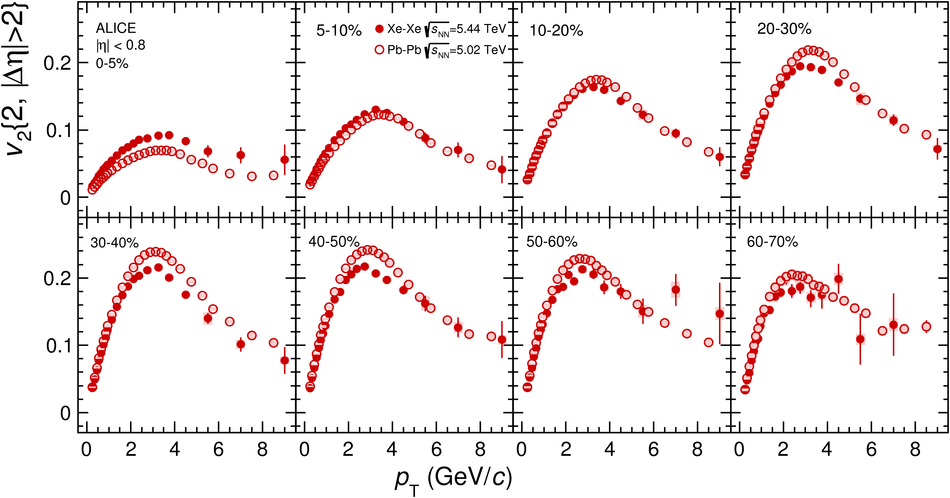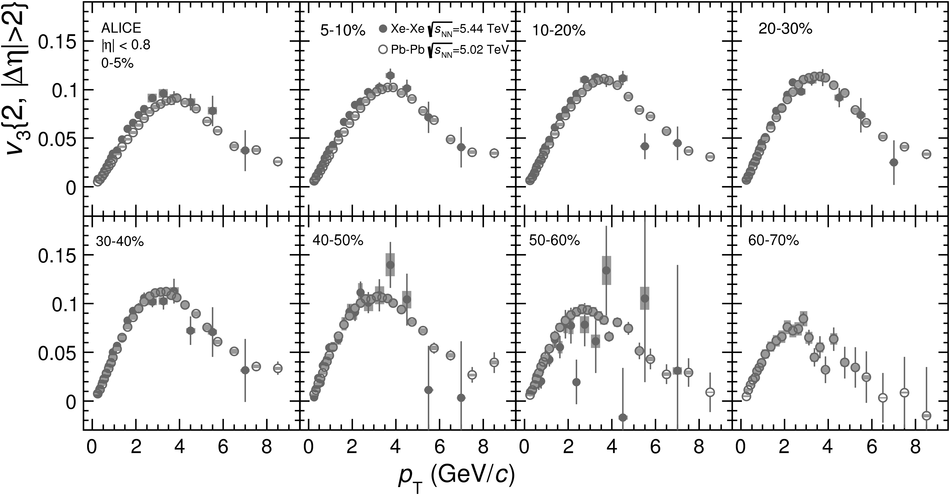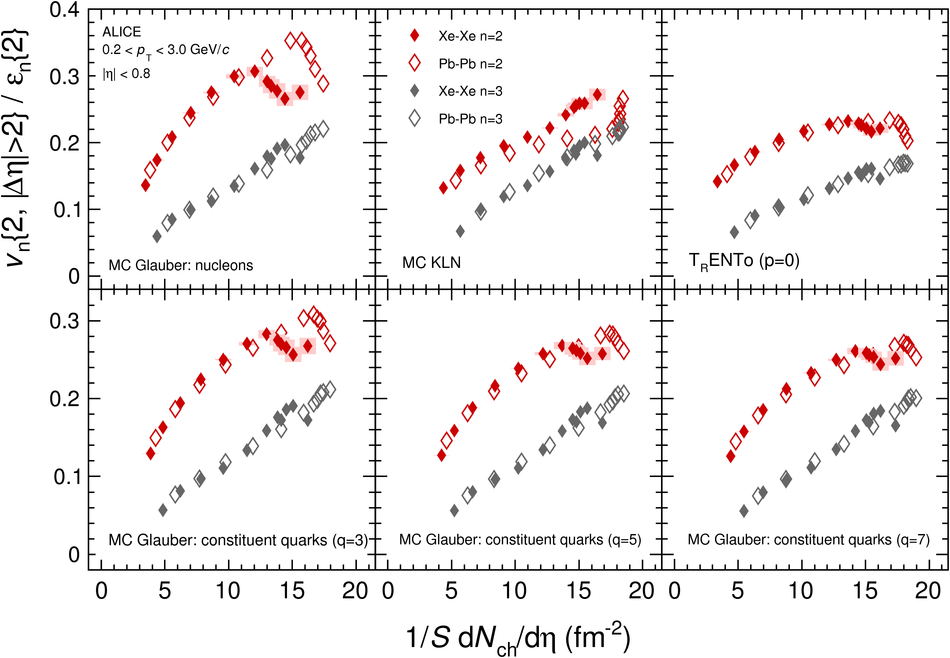# Anisotropic flow in Xe-Xe collisions at $\mathbf{\sqrt{s_{\rm{NN}}} = 5.44}$ TeV

The first measurements of anisotropic flow coefficients $v_{\rm{n}}$ for mid-rapidity charged particles in Xe-Xe collisions at $\sqrt{s_{\rm{NN}}} = 5.44$ TeV are presented. Comparing these measurements to those from Pb-Pb collisions at $\sqrt{s_{\rm{NN}}} = 5.02$ TeV, $v_{2}$ is found to be suppressed for mid-central collisions at the same centrality, and enhanced for central collisions. The values of $v_{3}$ are generally larger in Xe-Xe than in Pb-Pb at a given centrality. These observations are consistent with expectations from hydrodynamic predictions. When both $v_{2}$ and $v_{3}$ are divided by their corresponding eccentricities for a variety of initial state models, they generally scale with transverse density when comparing Xe-Xe and Pb-Pb, with some deviations observed in central Xe-Xe and Pb-Pb collisions. These results assist in placing strong constraints on both the initial state geometry and medium response for relativistic heavy-ion collisions.

Figures

## Figure 1

 Top panel: Charged particle $v_{\rm n}$ integrated over the transverse momentum range $0.2< p_{\rm{T}} < 3.0$~GeV/$c$ as a function of centrality from Xe--Xe collisions. The various techniques are explained in the text. Only statistical uncertainties are visible (thin vertical lines). Bottom panel: Ratios of $v_{2}\{4\}$/$v_{2}\{2\}$ compared to some theoretical predictions. The hydrodynamic predictions use a shear viscosity over entropy ratio $\eta/s=0.047$ and initial conditions from the T$_{\rm{R}}$ENTo model . For $v_{2}\{2\}$, the ALICE measurements implement a $|\Delta\eta|>2.0$ gap which is not used in the models.## Figure 2

 Top panel: Comparisons of charged particle $v_{\rm n}\{2\}$ integrated over the transverse momentum range $0.2< p_{\rm{T}} < 3.0$~GeV/$c$ as a function of centrality from Xe--Xe and Pb--Pb collisions. Middle panel: Ratio of $v_{\rm n}\{2\}$(Xe--Xe/Pb--Pb) coefficients. Bottom panel: Double ratio of data and theory. Hydrodynamical model predictions from EKRT and V-USPHYDRO are shown. In all cases, only statistical uncertainties are visible (thin vertical lines).## Figure 3

 Top panel: Comparisons of charged particle $v_{\rm n}\{2\}$ integrated over the transverse momentum range $0.2< p_{\rm{T}} < 3.0$~GeV/$c$ from Xe--Xe and Pb--Pb collisions for finer centrality bins in central collisions. Statistical and systematic uncertainties are shown as lines and boxes, respectively. Bottom panel: Corresponding ratio of $v_{\rm n}\{2\}$(Xe--Xe/Pb--Pb) coefficients.## Figure 4

 The $p_{\rm{T}}$-differential $v_{2}$ for charged particles from \sNNxs and \sNNps for various centrality classes. Statistical and systematic uncertainties are shown as lines and boxes, respectively.## Figure 5

 The $p_{\rm{T}}$-differential $v_{3}$ for charged particles from \sNNxs and \sNNps for various centrality classes. Statistical and systematic uncertainties are shown as lines and boxes, respectively.## Figure 6

 Comparisons of $v_{\rm{n}}\{2\}/\varepsilon_{\rm{n}}\{2\}$ integrated over the transverse momentum range $0.2< p_{\rm{T}} < 3.0$~GeV/$c$ as a function of $1/S$ d$N_{\rm{ch}}$/d$\eta$ in Xe--Xe and Pb--Pb collisions, where $S$ and $\varepsilon_{\rm{n}}\{2\}$ are from various initial state models . The models are explained in the text. The $^{129}$Xe deformation implemented is $\beta_{2}=0.18\pm0.02$, with the box errors representing the uncertainty in $\beta_{2}$. Measurements of d$N_{\rm{ch}}$/d$\eta$ ($|\eta|< 0.5$) from Xe--Xe and Pb--Pb collisions were obtained from separate studies .## Figure 7

 Top panel: Comparison of $p_{\rm{T}}$-differential $v_{\rm{2}}\{2\}/\varepsilon_{\rm{2}}\{2\}$ from Xe--Xe and Pb--Pb collisions for a selection of centrality bins. Statistical and systematic uncertainties are shown as lines and boxes, respectively. Bottom panel: Ratios of the scaled coefficients from the top panel. The Pb--Pb points are interpolated in order to determine the ratio. The circle markers show Xe--Xe 20--30\%/Pb--Pb 30--40\% while the square makers show Xe--Xe 30--40\%/Pb--Pb 30--40\%.